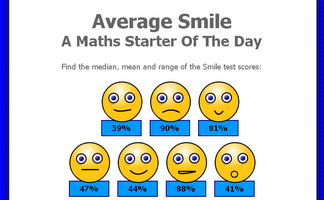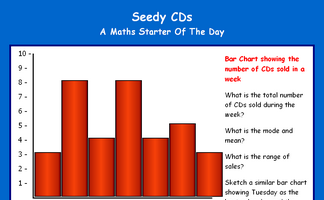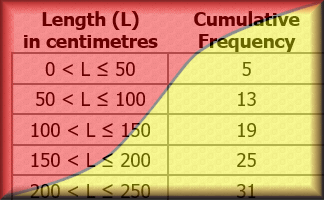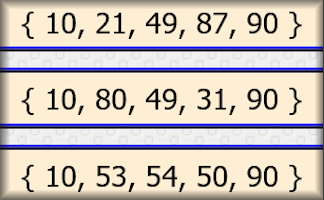#There are 366 different Starters of The Day, many to choose from. You will find below some starters on the topic of Averages. A lesson starter does not have to be on the same topic as the main part of the lesson or the topic of the previous lesson. It is often very useful to revise or explore other concepts by using a starter based on a totally different area of Mathematics.

Main Page

### Averages Starters:Calculate the mean, median and range of the given scores.Work out the number of chin ups the characters do on the last day of the week give information about averages.Five digits have the same mean, median, mode and range.The numbers on five houses next to each other add up to 70. What are those five numbers?Interpret data given in the form of a bar chart then create a new bar chart showing the given statistics.

## Exercises#### Averages

Test your understanding of averages with this self marking quiz about mean, median and range.#### Box Plots

An exercise on reading and drawing box-and-whisker diagrams which represent statistical data.This is a game for two players. You should know how to find the mean, median and range of a set of numbers.#### Cumulative Frequency

Online exercises on cumulative frequency for discrete and grouped data with exam-style questions.#### Standard Deviation

Find how 'spread out' data is by estimating and calculating this measure of dispersion.

### Search

The activity you are looking for may have been classified in a different way from the way you were expecting. You can search the whole of Transum Maths by using the box below.

Have today's Starter of the Day as your default homepage. Copy the URL below then select
Tools > Internet Options (Internet Explorer) then paste the URL into the homepage field.

Set as your homepage (if you are using Internet Explorer)

Do you have any comments? It is always useful to receive feedback and helps make this free resource even more useful for those learning Mathematics anywhere in the world. Click here to enter your comments.For All: